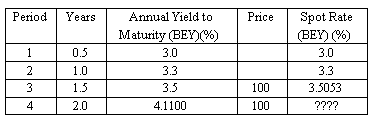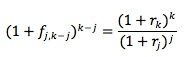Fixed Income I

Reading 44. Introduction to Fixed-Income Valuation

Learning Outcome Statements

i. define and compare the spot curve, yield curve on coupon bonds, par curve, and forward curve;

j. define forward rates and calculate spot rates from forward rates, forward rates from spot rates, and the price of a bond using forward rates;

CFA Curriculum, 2020, Volume 5

### Why should I choose AnalystNotes?

AnalystNotes specializes in helping candidates pass. Period.

### Subject 7. The Maturity Structure of Interest Rates

A yield curve is typically constructed on the basis of observed yields and maturities. There are different types of yield curves.The most common type is the upward-sloping yield curve. The longer maturity issues have higher yields than the shorter maturity issues.

A spot rate is the yield on a zero-coupon bond. A series of spot rates (spot curve) can be used to discount the cash flows of a bond.

Default-free spot rates can be derived from the Treasury par yield curve by a method called bootstrapping. The basic principle of bootstrapping is that the value of a Treasury coupon security should be equal to the value of the package of zero-coupon Treasury securities that duplicate the coupon bond's cash flows.

Example

Determine the spot rate for the fourth period cash flow. The coupon rate is 4.11%, paid semi-annually.The coupon for each period should be discounted at the corresponding spot rate.

100 = 2.055/(1+0.03/2)1 + 2.055/(1+0.033/2)2 + 2.055/(1+0.035053/2)3 + 102.055/(1+i/2)4 = 2.0246 + 1.9888 + 1.9506 + 102.055/(1+i/2)4

i = 2.0669% and the annualized spot rate is 4.1339%.

A par curve is a sequence of yields-to-maturity in which each bond is priced at par value. A par curve is obtained from a spot curve. All bonds used to derive the par curve are assumed to have the same credit risk, periodicity, currency, liquidity, tax status, and annual yields.

A forward rate refers to the interest rate on a loan beginning some time in the future. In contrast, a spot rate is the interest rate on a loan beginning immediately. For example, the two-year forward rate one year from now is 4%. This means that if you borrow a two-year loan one year from now, you will pay an interest of 4%.

A forward curve is a series of forward rates, each with the same time frame.

Forward rate calculations are usually based on a theoretical spot rate curve. They are sometimes referred to as implicit forward rates.

Given spot rates for maturities of j and k years, you can compute the forward rate (fj, k-j) that applies for the period from year j to year k using the relationship:Example

Compute the annualized six-month forward rate two years from now.In order to compute the six-month forward rate two years from now, first determine the spot rate for the fifth period (0.087) and the spot rate for the fourth period (0.0700). Then complete the following calculation: [(1 + 0.0875/2)5/(1 + 0.0700/2)4] - 1 = 7.95%.

Similarly, implied spot rates can be calculated as geometric average of forward rates.

###### kalps

THIS IS RUBBISH AND WORRYING AS YOU DIVIDE THE SPOT RATES BY TWO BUT THEY KEEP THE POWERS THE SAME - SURELY THEY SHOULD BE DOUBLED TO REFLECT THE FACT THAT YOU ARE LOOK AT SEMI ANNUAL PERIODS - CAN SOMEONE CONFIRM THIS IS TRUE IT WHETHER I AM TALKING RUBBISH ??

###### shasha

sorry kalps, i believe you got some concepts wrong here. 1st, spot rates are always annually quoted. 2ndly, when you see "1f14" referring to six month forward rate (of course 14 periods from now), you'd understand "one period" is six months, and 14 periods is 7 years. when "1f14" is calculated, all calculation must be based on six-month interval, so spot rate is divided by 2, as per the powers, they're already "doubled", say, from 7 (years) to 14 (intervals), so powers are kept unchanged. re the mistake, the answer did got one: question is asking for 1f14, it should be 7.72%. Should annualized rate be asked, the answer would be 15.44% then.

###### theLogic

Shasha: the answer is correct: it has the same numbers as you indicate. So what's wrong with it?

###### kkevin77

Does anybody have a quick and dirty answer to construct the forward yield curve? I'm trying to calculate the 10-yr treasury two years from now?

###### Treynor

I don't know what maturities that are available for Treasuries (since I am not living in US) but the calculation is pretty straightforward.

today's yield on 2-year^2 * future(2years from now) 10-year^10 = today's 12-year^12

You have the 2-year yield and if you don't have any 12-year you will have to approximate this one. For example through interpolation between a 10 year and a longer bond. After that you simply solve for the forward 10-year yield.

###### synner

all forward rates are reported in annual rates just like spot rates. so need to multiply it by 2 to get the final answers. 15.446% is correct.

###### NillePet

The assumption when calculating forwrad rates is that there are no abitrage possibilties. Thus, investing \$100 for 15 periods must have the same return as investing \$100 for 14 periods + reinvest the outcoming for another period.

Here we have a return for 15 periods of:
100 * (1+0.056139/2)^15 = 151,54

The return for 14 periods is:
100 * (1+0.04929/2)^14 = 140,52

Thus the forward rate 1f14 is:
(151,40-140,52)/140,52 = 7,84 %

This is equal to: (1+0.056139/2)^15/(1+0.04929/2)^14

So, I believe that 15,446% is wrong. If you search on google for forward rate calculations / definitions you find my caculation confirmed.
(1+0.056139/2)^15/(1+0.04929/2)^14

###### zackychoo

Is the convention of converting semi-annual to annual rates assumed in the exam? That is, do we definitely multiply or divide by 2 instead of squaring and rooting?

###### SeanDec10

NillePet - you did not carry enough decimal places in your calculation and are suffering from round-off error.

###### johntan1979

Sounds like kalps is referring to himself

###### floydbite

hey in the first example how did they get i=2.6609%??? I got i=4.1339% directly. And do we have to annualize i (*2) or just i is directly the answer?

###### vfabianv

Hello floybide, how did you get the i=4.1339% directly?

###### BIDA11

Is there a way to quickly calculate this on the BA II Plus calculator? Also how did you derive the interest of 102.055/(1+i/2)4 , i = 2.0669%, i cant seem to get my head around this. Thanks

###### amingraham

Calculate
FV = 102.055
PV = 100 - 2.0246 - 1.9888 - 1.9506 = 94.036
PMT = 0
N = 4
CPT I/Y = 2.0669

###### phill

amingraham, how did you get 102.055 for FV? Also, in the answer, they use 2.055 as the PMT, where does that come from? Thanks!